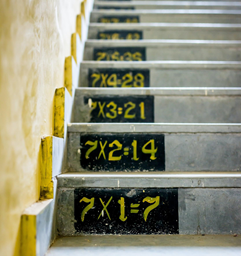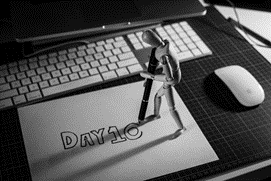Mathematics

# Why Is Math So Hard?

993 views

 1 Introduction 2 Why do people hate math? 3 Why is math so hard? 4 Summary 5 About Cuemath 6 FAQs 7 External references

8 Jan 2021

## Introduction

M-A-T-H-S. The very word conjures an image of numbers and confusion. Such is the fear of Maths that even the Maths teachers look stern and are usually unsmiling and unrelenting too. A stern look from them can scare the most talkative student in class into a frozen silence!

The moment a Maths teacher enters the class there is usually a revered silence with the message going across to every student- “this subject is not FUN and EASY it is MATHS and only the super-intelligent can score here.

One thought is persistent in the students’ heads “Why is math so hard?”Their hype around this subject is such that your brilliance in art, languages, and humanities is considered pathetic if you do not score in Maths! Science subjects follow Maths dutifully and we are told that if you are not good in Maths then the haloed portals of scientific temper are also denied to you.

So, the reason you think “why is math so hard for me” is because you have heard it so many times that without even trying you believe that Math is hard. Download the PDF to understand  “Why do people hate math?”

## Why do people hate math?

Another myth is that you need to be the “Maths type” then Maths is not so hard for you.

Strangely this typecasting is reserved only for Maths. Nobody eulogizes a student as “a History” type “a Hindi” type etc. This typecasting is reserved for Maths because Math is hard!Notwithstanding this social status accorded to Maths, one needs to understand the dichotomy between “scores” and a Mathematical temper.

If the teachers focused more on developing a Mathematical temper and forgot about the “scores”, they would do yeoman’s service to the souls of the great Mathematicians who walked this earth.

## Why is Math so hard?

If we were to conduct a survey, we would easily have at least 70% of students support the belief that “ “Why is Math so hard?”. Keeping this situation in mind, we have a lot of thinking to do. To begin with, let us understand why is Math so hard?

I think we look at numbers in a very abstract way. So, to begin with, let us make Maths look human. Have you ever tried looking at Maths like a language? A mode of communication? Do you find this idea bizarre?Well, Galileo did not he said: “the laws of nature are written in the language of Mathematics” Which means that the language of Mathematics has been around for a long time, even before language.

Let me give you an example of “why is math so hard”. Here is a question that was given to a 3rd Grade Maths:

How much would you spend - ½ on alpha and ¼ on beta and what would you be left with?

I can bet that most students in class-3 would be :Hello? Who is alpha? Who is beta? Can the problem be humanized, please? Use language and rewrite the problem with identifiable objects in place of alpha and beta.

We need to rework the way we ask students questions in Maths. We have to help them with illustrations to understand how a certain formula is arrived at. Better still the teacher needs to motivate the students with illustrations and real-life examples to arrive at the formula themselves.

So, make Concepts in Maths experiential for the student. Students find concepts in Maths very abstract.

Use the internet or use tools to explain the concepts clearly to the student. Trust me nothing can match this Eureka moment when a child discovers a formula!Another perception is that using fingers to count is babyish or childish. There are studies that prove that when students use fingers, they perform Maths problems much better. In other words, a number becomes more real and less abstract for the learner.

Neuroscientists support and recommend that students use fingers for counting.

Another reason why students end up struggling with Math is that they don’t understand the language of the problem. The information given is muddled and the students are not able to draw a mental picture.

Here the teachers and parents can illustrate the problem using diagrams or objects. They also need to encourage the student to get into the habit of illustrating problems.Sometimes you need extra support and that is not accessible. This is another reason why students end up struggling with Math. Teachers need to encourage collaborative learning so that students support each other and learn concepts or understand problems in a non-threatening environment.

Another reason why Math is hard especially for students go to high school is the emphasis on “pass marks” The question papers set by the teachers need to be mapped to concepts and skills to be used by the student. In this way, the teacher gets a clear picture or map of how well a student has understood a concept.

This will in turn help the teacher to revisit that concept and work on additional exercises related to the concept to make the student thorough. Most students find topics like Algebra, trigonometry, and Calculus confusing because they might have problems with some foundational principles of Mathematics.An analysis conducted in a class where students found Algebra difficult was traced to the discovery that the students only knew about 45% of Multiplication facts. These children are the ones who repeatedly believe that math is hard and the question “Why is math so hard for me?”.

Teachers and Parents need to shed their obsession with accuracy and allow students to learn by making mistakes. Have you ever considered the truth in the age-old adage that we learn best from mistakes? They why is this revelation not applied to Mathematics?

Maybe this is is worth noting down for a huge concern like “why is math so hard?”

As educators, we need to change the message that is going out to the students. Give the students space and tools to learn by themselves or collaboratively. Give them the time and space to learn through hit and trial.

As educators, your focus needs to be on the learning outcome and not berate the child on mistakes. And try to remove this thought in their head of math is hard.

## Summary

To get the thought that Math is hard, out of the minds of the students. We need to focus on why Math is so hard and what can educators do about it?Unless we get Maths out of the straight jacket and cheerless classrooms and even worse the didactic Maths labs, Maths will continue to be a dreaded subject and haunt the students with a morbid fear of examinations and scores and concrete the thought that math is hard.

It’s time we brought about a revolution in the way we teach Mathematics!

Cuemath, a student-friendly mathematics and coding platform, conducts regular Online Live Classes for academics and skill-development, and their Mental Math App, on both iOS and Android, is a one-stop solution for kids to develop multiple skills. Understand the Cuemath fee structure and sign up for a free trial.

Written by Jayashree Nair

## Why is math so hard?

So, Math is hard because you have heard it so many times that without even trying you believe that Maths is hard.

## Why do people hate math?

Another myth is that you need to be the “Maths type” then Maths is not so hard for you.

Strangely this typecasting is reserved only for Maths. Nobody eulogizes a student as “a History” type “a Hindi” type etc. This typecasting is reserved for Maths because Math is hard!

Notwithstanding this social status accorded to Maths, one needs to understand the dichotomy between “scores” and a Mathematical temper.

If the teachers focused more on developing a Mathematical temper and forgot about the “scores”, they would do yeoman’s service to souls of the great Mathematicians who walked this earth.

## External references

Please click below to know more:

Why is math so hard

Why High School Students Feel Mathematics Difficult?

Award-winning math curriculum, FREE for a year